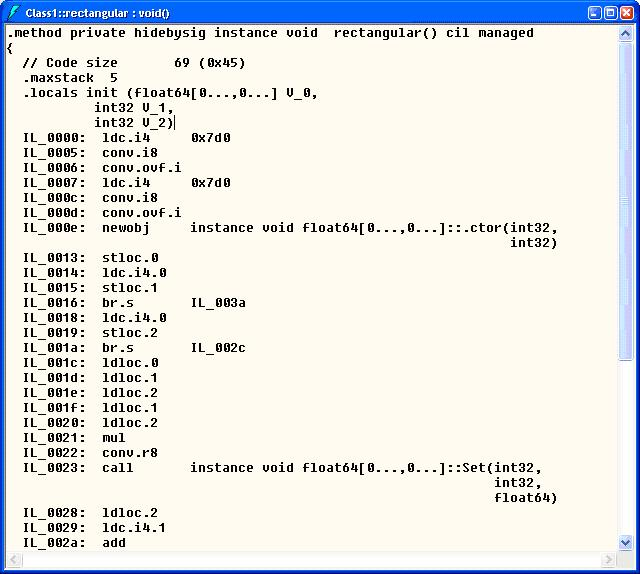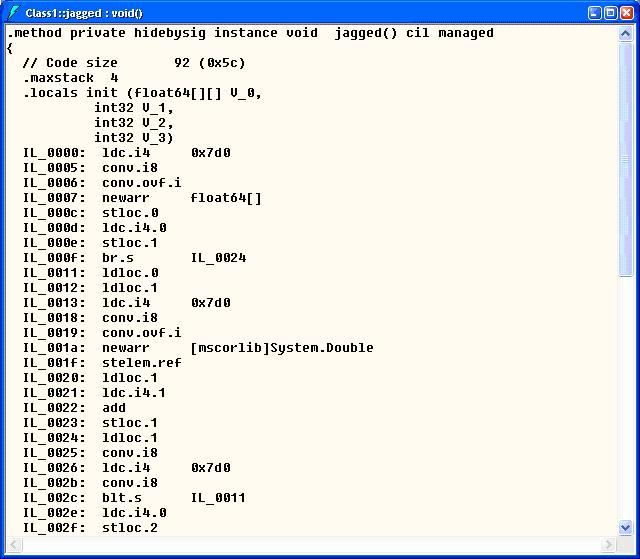## Performance Comparison: Rectangular Array vs. Jagged Array

Jagged arrays (e.g. a[i][j]) are named such so because it is an array of array. Because each dimension within an array can be different for different indices, jagged arrays can be much more efficient in terms of the memory usage in certain scenarios such as sparse matrices manipulation when compared to rectangular arrays. But the perception of the rectangular array (a continuous block of memory) has somehow suggested its superior performance comparing to a jagged array.

This, however, is not true within the .Net Framework (the analysis herein is based on .Net Framework 1.1). And synthetic tests suggest that we should use jagged arrays whenever it is possible, since it is always superior to it’s counterpart in almost every way (both speed and memory usage). Even when used as a full rectangular array, jagged arrays still have some performance advantages to rectangular array. Here is a concrete test I did.

In order to measure the performance of each of the methods, we created an array of 40000000 elements. And we measure how fast it is to populate these two arrays.

const long dimension = 2000; void jagged() { double[][] a; a = new double[dimension][]; for (int i = 0 ; i < dimension ; i ++) a[i] = new double[dimension]; for (int i = 0 ; i < dimension; i ++) for (int j = 0 ; j < dimension ; j++) a[i][j] = i*j; } void rectangular() { double[,] a = new double[dimension,dimension]; for (int i = 0 ; i < dimension; i ++) for (int j = 0 ; j < dimension ; j++) a[i,j] = i*j; }

The result shows that the jagged array performed roughly 12% better then the rectangular array. It took 56 ms to execute the jagged() while it took 63 ms to execute rectangular(). I will explain how the time is measured in some later blogs. So, why is jagged array more efficiently in .Net? Maybe we can find out some clues from the ILs the code generates.It seesms that we have found our answer. In the ILDASM screen for the rectangular array method, we saw that the two dimensional array was treated as a whole object:

IL_000e: newobj instance void float64[0…,0…]::.ctor(int32, int32)

Whereas in the IL generated for the jagged array method, the array is treated differently:

IL_0007: newarr float64[] IL_001a: newarr [mscorlib]System.Double

And I suspect that that’s where the efficiency comes from. The conclusion is, jagged array performs superior than rectangular array in .Net framework 1.1 (I will perform some analysis in 2.0 later), so we should use jagged array instead of rectangular arrays whenever we can.